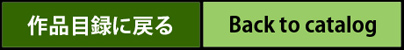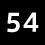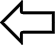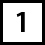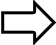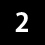The Vast Universe1961　aproduct graph HARUO KANEKO　age14 100cm×66cm　Oil Color The diameter of the universe10×26th power（m） The number of electrons in the whole universe 10×87th power End of the universe 15 billion light years = 15,000,000,000 light years A light-year 9.46 × 10×15th power（m） = 9,460,000,000,000,000 m = Km m = 9,460trillion m.= 9trillion 4,600billion km.( 10 trillion km) 1light-second 299,000km. The number of galaxies is more than 10 billion Nebulae there more than 10 billion ~ 100 billion in the universe. The diameter of our galaxy (Milkyway) 9 × 10×20th power（m）　 (Ly 10-150000) about 100quintillion km. Our galaxy also homes some one hundred thousand nebulae. The number of the stars of the whole universe 10×22th power From earth to sun distance 149,6000,0000 km. 1.27 × 107m = 11,780,000x diameter of the earth = 12,700 km The Diameter of the Earth: 1.27 × 10×7th power（m） = 12,700,000m = 12,700km Equatorial Radius = 6,378km. Pole Radius = 6,356km. The Diameter of the Sun 1.39 × 10×9th power（m） = 1,390,000,000m. = 139million km. The Radius of the sun is equal to 109 x the radius of the Earth = 696,000km. The mass of the Sun is 1,989x10×30th power（kg）= 333,000 times the mass of Earth. Black Holes: Gravity is too strong for high-density also celestial bodies cannot emit light as well. The Big Bang Theory: the beginning of time space explosion, it is therefore necessary to look or think contrary to this reason. This is because it is not supposed to Ru by the explosion, the mathematical order of the universe is generated The Moon, Diameter= 3476km. Radius= 1738km one quarter of the Earth. Gravity = one sixth of earth The mass of the Earth is one 81th of the earth. The Volume is 1 fiftieth of earth. The average distance from earth is 384,400 km, 30 times the diameter of the Earth. Note: The above was written, the number of the diameter of the universe, the whole universe of electrons, the end of the universe, star of the whole universe There is only one theory of the world, of a limited range, is that of the universe, the number of, that is, human I only universe that can be observed in the range. It is located in the organism compared to one of the cell Is the. However, much more, in fact, as a three-dimensional world, the whole universe is vast than that Is, we can not understand in any way, is a vast Muhen, a human, that is, the numbers in Table I Is no other than the thing can not be said to nest, and infinity. (∞)